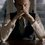# Another Calculus Challenge!

Prove the following identity -

$\displaystyle \prod_{r=1}^{n}{\Gamma\left(\frac{r}{n+1}\right)} = \sqrt{\frac{{(2\pi)}^{n}}{n+1}}$

Please be original. I hope you will not copy from sites like MSE(where it might be discussed).Note by Kartik Sharma
5 years, 2 months ago

This discussion board is a place to discuss our Daily Challenges and the math and science related to those challenges. Explanations are more than just a solution — they should explain the steps and thinking strategies that you used to obtain the solution. Comments should further the discussion of math and science.

When posting on Brilliant:

• Use the emojis to react to an explanation, whether you're congratulating a job well done , or just really confused .
• Ask specific questions about the challenge or the steps in somebody's explanation. Well-posed questions can add a lot to the discussion, but posting "I don't understand!" doesn't help anyone.
• Try to contribute something new to the discussion, whether it is an extension, generalization or other idea related to the challenge.

MarkdownAppears as
*italics* or _italics_ italics
**bold** or __bold__ bold
- bulleted- list
• bulleted
• list
1. numbered2. list
1. numbered
2. list
Note: you must add a full line of space before and after lists for them to show up correctly
paragraph 1paragraph 2

paragraph 1

paragraph 2

[example link](https://brilliant.org)example link
> This is a quote
This is a quote
    # I indented these lines
# 4 spaces, and now they show
# up as a code block.

print "hello world"
# I indented these lines
# 4 spaces, and now they show
# up as a code block.

print "hello world"
MathAppears as
Remember to wrap math in $$ ... $$ or $ ... $ to ensure proper formatting.
2 \times 3 $2 \times 3$
2^{34} $2^{34}$
a_{i-1} $a_{i-1}$
\frac{2}{3} $\frac{2}{3}$
\sqrt{2} $\sqrt{2}$
\sum_{i=1}^3 $\sum_{i=1}^3$
\sin \theta $\sin \theta$
\boxed{123} $\boxed{123}$

Sort by:

Lemma :

$\prod_{r=1}^{n-1}\sin\left(\dfrac{r\pi}{n}\right)=\dfrac{n}{2^{n-1}}$

Proof:

$\because\sin(x) = \frac{1}{2i}(e^{ix}-e^{-ix})$

$\displaystyle\implies\prod_{k=1}^{n-1} \sin\left(\dfrac{k\pi}{n}\right) = \left(\dfrac{1}{2i}\right)^{n-1}\prod_{k=1}^{n-1} \left(e^{\frac{k\pi i}{n}} - e^{\frac{-k\pi i}{n}}\right)$

$\displaystyle= \left(\dfrac{1}{2i}\right)^{n-1} \ \left(\prod_{k=1}^{n-1} e^{\frac{k\pi i}{n}} \right) \prod_{k=1}^{n-1} \left(1-e^{\frac{-2k\pi i}{n}} \right)$

$\displaystyle= \left(\dfrac{1}{2i}\right)^{n-1} \times i^{n-1} \prod_{k=1}^{n-1} \left(1-e^{\frac{-2k\pi i}{n}} \right)$

$\displaystyle= \dfrac{1}{2^{n-1}} \prod_{k=1}^{n-1} \left(1-e^{\frac{-2k\pi i}{n}} \right)$

Consider the factorisation,

$x^{n-1}+x^{n-2}+ \ldots + x + 1 = \prod_{k=1}^{n-1}\left(x-e^{\frac{-2\pi k}{n}}\right)$

Putting $x=1$ in the above identity gives,

$\displaystyle \prod_{k=1}^{n-1} \left(1-e^{\frac{-2k\pi i}{n}} \right)=n$

$\displaystyle \therefore \prod_{r=1}^{n-1}\sin\left(\dfrac{r\pi}{n}\right)=\dfrac{n}{2^{n-1}}$

Now, consider

$\text{S}=\sum_{r=1}^{n}\log \left(\Gamma{\left(\dfrac{r}{n+1}\right)}\right)$

$\displaystyle = \sum_{r=1}^{n}\log \left(\Gamma{\left(\dfrac{n+1-r}{n+1}\right)}\right) \ \left(\because \sum_{r=a}^{b}f(r)=\sum_{r=a}^{b}f(a+b-r)\right)$

$\displaystyle = \sum_{r=1}^{n}\log \left(\Gamma{\left(1-\dfrac{r}{n+1}\right)}\right)$

By Euler's Reflection Formula,

$\displaystyle \text{S} = \sum_{r=1}^{n}\log \left(\dfrac{\pi}{\Gamma{\left(\dfrac{r}{n+1}\right)}\sin \left(\dfrac{r\pi}{n+1}\right)}\right)$

$\displaystyle =\log ({\pi}^n) - \sum_{r=1}^{n}\log\left(\Gamma{\left(\dfrac{r}{n+1}\right)}\right) - \sum_{r=1}^{n} \log \left(\sin \left(\dfrac{r\pi}{n+1}\right)\right)$

$\displaystyle \implies 2\text{S} = \log({\pi}^n) - \sum_{r=1}^{n} \log \left(\sin \left(\dfrac{r\pi}{n+1}\right)\right)$

Using the Lemma, we have,

$2\text{S}=\log({\pi}^n) - \log\left(\dfrac{n+1}{2^n}\right)$

$\displaystyle \implies \text{S} = \log \left(\sqrt{\dfrac{(2\pi)^n}{n+1}}\right)$

$\displaystyle \implies \sum_{r=1}^{n}\log \left(\Gamma{\left(\dfrac{r}{n+1}\right)}\right) = \log \left(\sqrt{\dfrac{(2\pi)^n}{n+1}}\right)$

$\displaystyle \implies \log\left(\prod_{r=1}^{n}\Gamma{\left(\dfrac{r}{n+1}\right)}\right) = \log \left(\sqrt{\dfrac{(2\pi)^n}{n+1}}\right)$

$\boxed {\therefore \displaystyle \prod_{r=1}^{n}\Gamma{\left(\dfrac{r}{n+1}\right)}=\sqrt{\dfrac{(2\pi)^n}{n+1}}}$

- 5 years, 1 month ago

Yep. That's correct! That is also the way I did it. I am curious to know if there is any other approach. Maybe using convolution theorem?

- 5 years, 1 month ago

Another way is to use the Multiplication Theorem. To prove the multiplication theorem, the limit definition of gamma function can be used.

- 5 years, 1 month ago

Oh! That's nice! I didn't know about it.

To prove the multiplication theorem, the limit definition of gamma function can be used.

I don't understand what's the limit definition?

- 5 years, 1 month ago

$\displaystyle\Gamma(z)=\lim_{n\to \infty}\dfrac{n^z n!}{z(z+1)(z+2)\ldots(z+n)}$

- 5 years, 1 month ago

Oh! Okay fine, I get it.

- 5 years, 1 month ago